# Frobenius matrix

(diff) ← Older revision | Latest revision (diff) | Newer revision → (diff)

companion matrix

For every polynomialthere are-matricessuch that the characteristic polynomial of,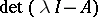, is equal to. Indeed, two such are: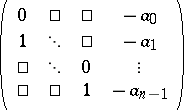(a1)

and(a2)

These two matrices are similar and their minimal polynomial (cf. Minimal polynomial of a matrix) is, i.e. their similarity invariants are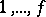(see Normal form). Both are called the companion matrix, or Frobenius matrix, of.

More generally, a matrix of block-triangular form with as diagonal blocks one of the companion matrices above (all of the same type),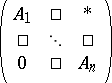is also sometimes called a Frobenius matrix.

Somewhat related, a matrix with just one column (or one row, but not both) different from the identity matrix is also sometimes called a Frobenius matrix; see, e.g., [a1], p. 169.

For the matrix (a1), the first standard basis vectoris a cyclic vector (see also Pole assignment problem). The vectors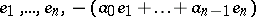form a so-called Krylov sequence of vectors for, that is, a sequence of vectors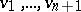such that,, the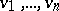are independent, and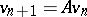is a linear combination of.

The first and second natural canonical forms of a matrix(see also Normal form) are block-diagonal with companion matrices as blocks. Both are also known as the Frobenius normal form of.

In a completely different setting, the phrase "Frobenius matrix" refers to a matrix giving the (induced) action of the Frobenius endomorphism of an algebraic variety of characteristic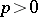on, say, the cohomology of that variety.

How to Cite This Entry:
Frobenius matrix. Encyclopedia of Mathematics. URL: http://encyclopediaofmath.org/index.php?title=Frobenius_matrix&oldid=12792
This article was adapted from an original article by M. Hazewinkel (originator), which appeared in Encyclopedia of Mathematics - ISBN 1402006098. See original article